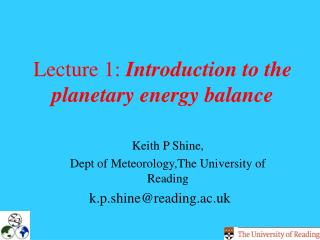# Lecture 1: Introduction to the planetary energy balance - PowerPoint PPT PresentationDownload PresentationLecture 1: Introduction to the planetary energy balance

Lecture 1: Introduction to the planetary energy balanceDownload Presentation## Lecture 1: Introduction to the planetary energy balance

- - - - - - - - - - - - - - - - - - - - - - - - - - - E N D - - - - - - - - - - - - - - - - - - - - - - - - - - -
##### Presentation Transcript

1. Lecture 1: Introduction to the planetary energy balance Keith P Shine, Dept of Meteorology,The University of Reading k.p.shine@reading.ac.uk

2. Structure • The solar “constant” – how much solar radiation is absorbed by the Earth • The effective emitting temperature of the Earth • Spectral variation of emission by the Sun and the Earth • A simple illustration of the energy balance and the greenhouse effect, including the stratosphere • A more realistic view of the energy balance • A few problems

3. Almost the only essential physics you will need to know ...... a “black body” (i.e. a perfect emitter/ absorber of radiation) emits a flux density (“irradiance”) = T4 (in Wm-2) into the hemisphere above it, averaged over all wavelengths.  is the Stefan-Boltzmann constant (5.67x10-8 Wm-2K-4) and T is the temperature in Kelvin.

4. The Solar Constant (1) • Solar Constant: somewhat dated term (now often referred to, more accurately, as the Total Solar Irradiance) which is defined as the number of Watts incident on a unit area perpendicular to a line joining the centres of the Earth and the Sun, at the mean Sun-Earth distance. • Measured So (from satellites) is about 1370 Wm-2 – implies Sun is emitting as a black body at a temperature, TSun, of about 5800 K. • Energy intercepted by Earth is So re2 where re is Earth’s radius .... but Earth has surface area of 4re2 ... Therefore …..

5. The Solar Constant (2) • Average solar irradiance incident on Earth is • But .... fraction of this incident radiation reflected back to space (by clouds, gases, aerosols and the surface)....this fraction is called the planetary albedo, p.Observations from satellites indicate that the value of p is about 0.3. • Hence, the total (rate of) solar energy absorbed by the Earth-atmosphere system is

6. Effective Emitting Temperature of the Earth (1) • At the top of the atmosphere there must be a long term balance between the energy absorbed from the Sun and the energy emitted to space by the Earth-atmosphere system. • Assume (but only for now!) that the Earth emits as a black body to space at a temperature Te (where the “e” could stand for Earth, or emitting, or effective!). In balance:

7. Effective Emitting Temperature of the Earth (2) • Note that Te is much less than the global average surface temperature, TS (about 288 K). • Now have two characteristic temperatures .. TSun=5800 K, and Te=255 K – can derive the variation of emitted radiation with wavelength from the Planck Function available in all good physics texts…. • Consequence is that, after “diluting” the emitted solar radiation as it spreads out from Sun, the solar radiation and the Earth emitted radiation (coincidentally!) are in separate wavelength regions separated at about 4 m … see Figure.

8. fig

9. Simple model of greenhouse effect (1) • Difference between Ts and Te is because of the greenhouse effect …water vapour, carbon dioxide, ozone, … and clouds absorb and emit thermal infrared radiation (i.e. at  > 4m). Simple illustration using single layer model of the atmosphere … following approximations: • Atmosphere does not absorb any solar radiation – all is absorbed at the Earth’s surface. • The atmosphere emits thermal infrared radiation as a grey body … it emits T4 upwards and downwards, where  is the emittance ( 1). Ability to emit and absorb are related so: emittance = absorptance and transmittance = 1 – absorptance. (e.g. for a black body =absorptance=1, transmittance=0). • The surface is a black body in the thermal IR.

10. Top of Atmosphere , Ta Ts Simple model of greenhouse effect (2) • Can show that • Note that if =0 (no absorbing atmosphere) then we get the earlier equation • “Pluck” a number from the air … =0.6, gives Ts=278 K … i.e. nearer observations. Increasing  (e.g. more CO2) increases Ts. • This simple equation encapsulates many of the “externally” forced climate change mechanisms .. changes in So,  and  can all cause climate change.

11. stTst4 S0/4 ast st, Tst stTst4 Why the stratosphere is different … • In the simple model above, the atmosphere is essentially heated from below as this is where the sun’s energy is deposited. • The stratosphere is different – it is heated from within, partly by absorption of solar radiation, ast, by ozone. A simple model of its energy balance is then: Notice that an increase in st (e.g. by increasing CO2) now leads to a cooling of the stratosphere, as is found in more sophisticated models

12. A more realistic energy budget • energy exchanges in the atmosphere are not solely radiative … surface fluxes of latent and sensible heat , vertical fluxes of latent and sensible heat by convection, fronts etc • the atmosphere does absorb some solar radiation • the value of  chosen earlier is entirely arbitrary – in reality the atmosphere is not a grey absorber – it absorbs well at some wavelengths, poorly at others (see next lecture).

13. Modern (global and annual averaged!) Earth energy budget from Kiehl and Trenberth (1997) FURTHER (INTRODUCTORY) READING Hartmann D.L. (1994) Global Physical Climatology, Academic Press Kiehl J.T. and K.E.Trenberth (1997), Earth’s Annual Global Mean Energy Budget. Bulletin of the American Meteorological Society, vol 78, 197-208.